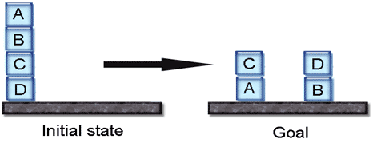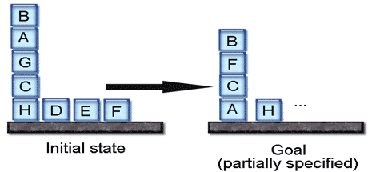### Introduction

PL-PLAN is an open-source ai planner written during the summer of 2004 by Philippe Fournier-Viger and Ludovic Lebel as a side project. The goal was to create a simple AI planner to experiment many search techniques for classical states-space exploration, including the Graphplan algorithm, and six algorithms based on partial-order theory. PL-PLAN can be used as a library from any Java programs. A software with a graphical user interface has been developed to run multiple random tests to compare the various algorithms of PL-PLAN. Tests results can be exported to comma-separated files that can be read by Microsoft Excel.### Theoretical Foundations

Our implementation of the Graphplan algorithm is based on the description found in this book : "M. Ghallab, D. Nau, and P. Traverso. Automated Planning: Theory and Practice. Morgan Kaufmann, May 2004"

Our partial order algorithms are directly inspired by the work done by P. Godefroid and M. Najjar:

• Godefroid, P. (1996). Partial-Order Methods for the Verification of Concurrent Systems -- An Approach to the State-Explosion Problem, Springer-Verlag, volume 1032 of Lecture Notes in Computer Science.
• Najjar, M. (2001). Planification par des Ordres Partiels. M. Sc. Dissertation. Department of Computer Science, University of Sherbrooke. Ref : Q 336 N34 2000 (Bibliothèque des Sciences).

The code of PL-PLAN is not fully optimized. It should be noted that the four partial-order algorithms based on persistant sets (algorithms with names prefixed by F1) do not always find optimal plans, because of our implementation of persistant sets .

### Source Code

The main class (PLPlan.java) is located in the "javaapi" package. We provided two examples (Example1.java, and Example2.java, described below on this page ). The first creates a new problem and then find a plan. The second load a problem definition from a file, and then find a plan. A couple of problem files are provided. They follow a format that we have defined and that is easily understandable. In the "gui" package there is the graphical interface that we use to launch automatic and manual tests. Although eight algorithms are available, we recommend using the Graphplan algorithm, as the others algorithms implementation are generally slower.

### Example 1

This example (Example1.java) shows how to create a new problem with PL-PLAN and find a plan.

### The problem

The problem is the classic Blockworld problem. There is four blocks on a table, as illustrated bellow. The Example1.java class utilize PL-PLAN to find a plan to go from the initial state to the goal state. A plan consists of applying a sequence of action. Each action is the application of an operator. In the blockworld problem, it is only possible to move one block at a time. For this example, we have only encoded the operators"uAB" (unstack A from B), "uBC" (unstack B from C), "uCD" (unstack C from D), "sCA" (stack C on A), "sDB" (stack D on B). In example 2, we use all the operators.### The Java Code

public class Example1 {

public static void main(String[] args) throws PlPlanException {

System.out.println(&quot;-- TEST1 --&quot;);<br />
// Create a planning problem, and then find a plan<br />
// This problem is based on Blockworld<br />
PLPlan planner = new PLPlan();<br />
planner.setAlgorithm(EnumAlgorithm.GRAPHPLAN);

// Facts (Initial state) //td, ocd, obc, oab, na

// Goal //( oca, odb, ta, tb, nc, nd)

// --- Operators ---
//        (op uAB
//                (na, oab)
//                (ta, nb)
//                (oab))
List<String> precond = new ArrayList<String>();
List<String> neg = new ArrayList<String>();
List<String> pos = new ArrayList<String>();

//      (op uBC     //   operator name : "uBC"  unstack block b from block c
//      (nb, obc)   //   predonditions : the b Block is on block c (obc) and
//                                       there is no block on block b (nb)
//      (tb, nc)    //   add facts :     the block b is on table (tb)
//                                       there is no block on block c (nc)
//      (obc))      //   remove facts : we remove the fact that
//                                      the b Block is on block c (ocd)
precond = new ArrayList<String>();
neg = new ArrayList<String>();
pos = new ArrayList<String>();

//        (op uCD             //   operator name : "uCD"  unstack block c from block d
//                (nc, ocd)   //   predonditions : the c Block is on block D (ocd) and
//                                                 there is no block on block c (nc)
//                (tc, nd)    //   add facts :     the block c is on table (tc)
//                                                 there is no block on block d (nd)
//                (ocd))      //   remove facts : we remove the fact that
//                                                the c Block is on block d (ocd)
precond = new ArrayList<String>();
neg = new ArrayList<String>();
pos = new ArrayList<String>();

//      (op sCA       // operator name : "sCA" stack block c on block a
//      (na, tc, nc)  // predonditions : there is no block on block a (na)
//                                       the block c is on table  (tc)
//                                       there is no block on block c (nc)
//      (oca)         // add facts :     the block c is on block a  (oca)
//      (na, tc))     // remove facts : we remove the facts that
//                                       - there is no block on block a (na),
//                                       - block c is on table (tc).
precond = new ArrayList<String>();
neg = new ArrayList<String>();
pos = new ArrayList<String>();

//      (op sDB       // operator name : "sDB" stack block d on block b
//      (nd, tb, nb)  // predonditions : there is no block on block d (nd)
//                                       the block b is on table (tb)
//                                       there is no block on block b (nb)
//      (odb)         // add facts :     the block d is on block b  (odb)
//      (nb, td))     // remove facts : we remove the facts that
//                                       - there is no block on block b (nb),
//                                       - block d is on table (td).
precond = new ArrayList<String>();
neg = new ArrayList<String>();
pos = new ArrayList<String>();

List resultats = planner.findPlan();
System.out.println(resultats);
}

}

### The Console Output

The solution : [uBA, uAG, uGC, uCH, sCA, sFC, sBF]

### Example 2

This example (Example2.java) shows how to load a problem from a file with PL-PLAN and then find a plan.

### The problem

The problem is the classic Blockworld problem. There is eight blocks on a table, as illustrated bellow. The Example2.java class utilize PL-PLAN to find a plan to go from the initial state to the goal state. A plan consists of applying a sequence of action. Each action is the application of an operator. In the blockworld problem, it is only possible to move one block at a time. For instance, in this example, "uAB" (unstack A from B), "uBC" (unstack B from C) and "sDB" (stack D on B) are operators.### The Java Code

PLPlan planner = new PLPlan();
planner = new PLPlan();
planner.setAlgorithm(EnumAlgorithm.FW_SS_SM_0);
List resultats = planner.findPlan();
System.out.println(resultats);

### The Console Output

The solution : [uBA, uAG, uGC, uCH, sCA, sFC, sBF]

If you use PL-PLAN, we ask you to cite our names in your work, and to send us an e-mail.This sofware is provided "as is", without warranty of any kind. The user takes the entire risk as to the quality and performance of the software. The authors accept no responsibility for any problem the user encounters using this software.

### Citations

The PL-PLAN software has been cited in the following publications:

• Tsao, T. Y.-T., Jih, W.-R., Hsu, J. Y.-J. (2012) MAPLE: multi-agent programming with letter exchanges on sensor networks. Proceedings of the 11th International Conference on Autonomous Agents and Multiagent Systems (AAMAS 2012). pp. 1439-1440.

• Hatzi, O. (2009). Web Service Composition through AI Planning. Ph.D. Thesis.

• Baki, B. (2006). Planification et ordonnancement probabilistes sous contraintes temporelles. Ph.D. Thesis.UNIVERSIT´E de CAEN/BASSE-NORMANDIE

• and others...